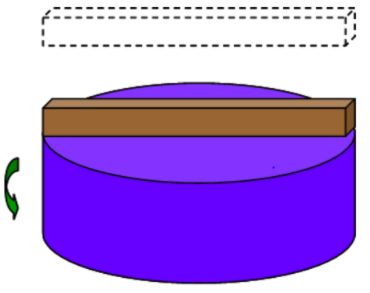# Problem: A solid disk of mass m1 = 9.1 kg and radius R = 0.18 m is rotating with a constant angular velocity of ω = 35 rad/s. A thin rectangular rod with mass m2 = 3.6 kg and length L = 2R = 0.36 m begins at rest above the disk and is dropped on the disk where it begins to spin with the disk.1).What is the initial angular momentum of the rod and disk system?2).What is the initial rotational energy of the rod and disk system?3). What is the final angular velocity of the disk?4).What is the final angular momentum of the rod and disk system?5).What is the final rotational energy of the rod and disk system?6)The rod took t = 5 s to accelerate to its final angular speed with the disk. What average torque was exerted on the rod by the disk?

###### FREE Expert Solution

1.

Angular momentum:

$\overline{){\mathbf{L}}{\mathbf{=}}{\mathbf{I}}{\mathbf{\omega }}}$ where I is the moment of inertia and ω is the angular velocity.

Moment of inertia of solid disk:

$\overline{){\mathbf{I}}{\mathbf{=}}\frac{\mathbf{1}}{\mathbf{2}}{\mathbf{M}}{{\mathbf{R}}}^{{\mathbf{2}}}}$

Moment of inertia of a rod:

$\overline{){\mathbf{I}}{\mathbf{=}}\frac{\mathbf{1}}{\mathbf{12}}{\mathbf{M}}{{\mathbf{R}}}^{{\mathbf{2}}}}$

The initial angular momentum of the rod is zero because its velocity is zero.

The initial angular momentum of the rod and disk system is the sum of the angular momentum of the two objects.

82% (343 ratings)###### Problem Details

A solid disk of mass m1 = 9.1 kg and radius R = 0.18 m is rotating with a constant angular velocity of ω = 35 rad/s. A thin rectangular rod with mass m2 = 3.6 kg and length L = 2R = 0.36 m begins at rest above the disk and is dropped on the disk where it begins to spin with the disk.1).What is the initial angular momentum of the rod and disk system?

2).What is the initial rotational energy of the rod and disk system?

3). What is the final angular velocity of the disk?

4).What is the final angular momentum of the rod and disk system?

5).What is the final rotational energy of the rod and disk system?

6)The rod took t = 5 s to accelerate to its final angular speed with the disk. What average torque was exerted on the rod by the disk?